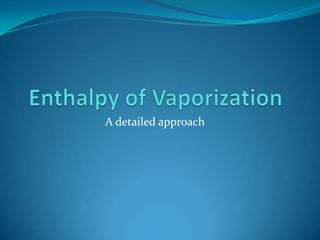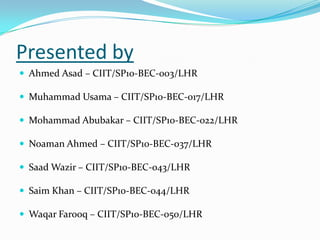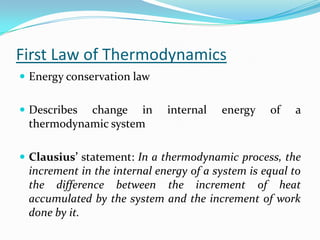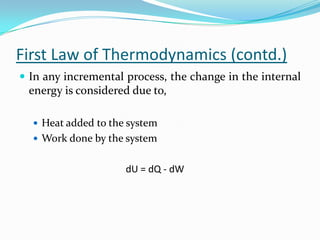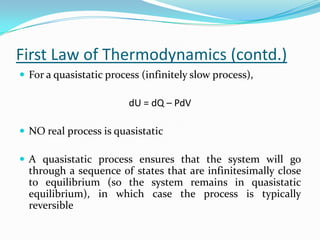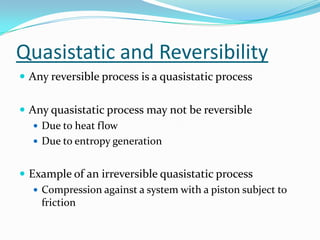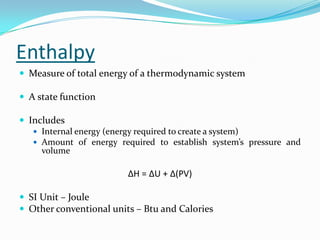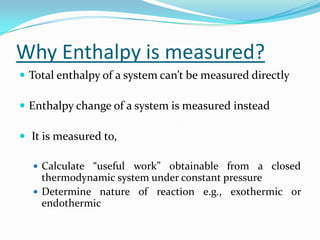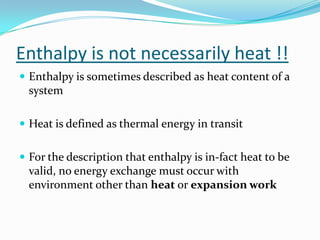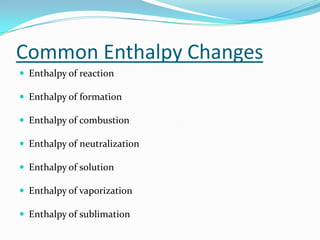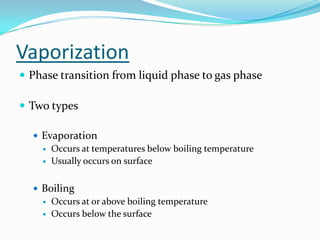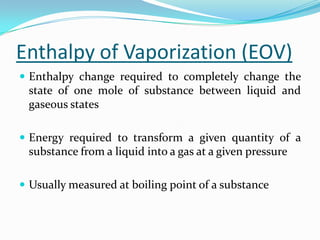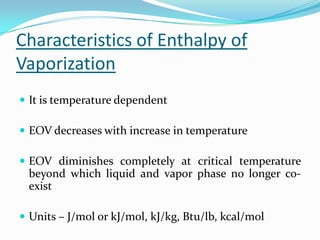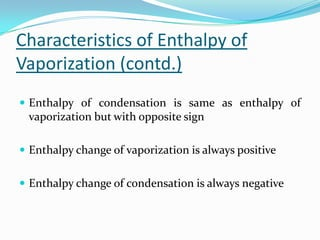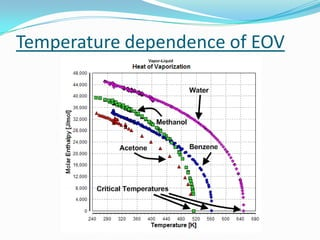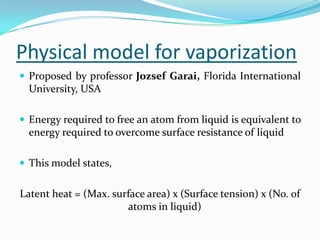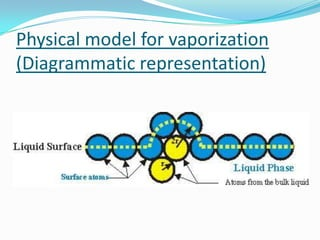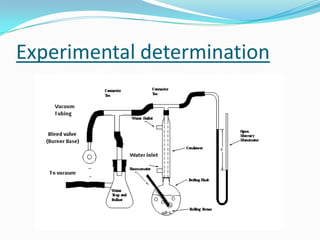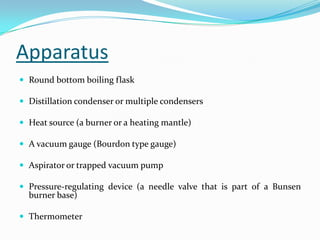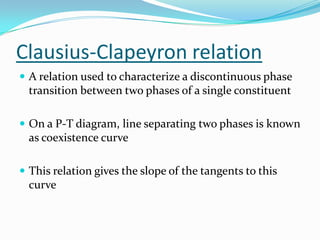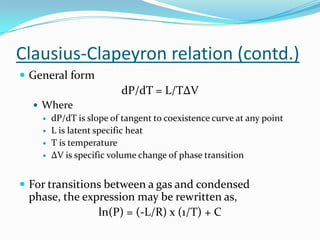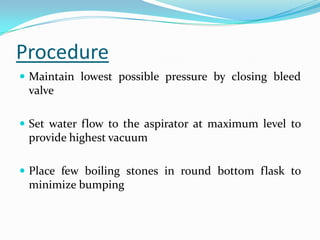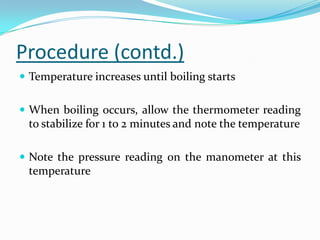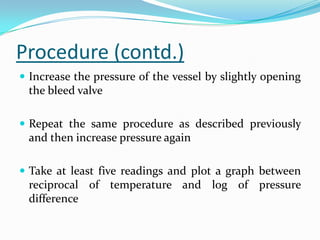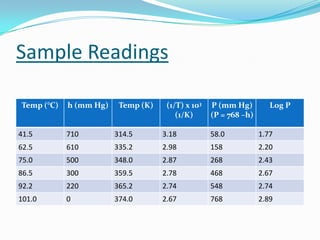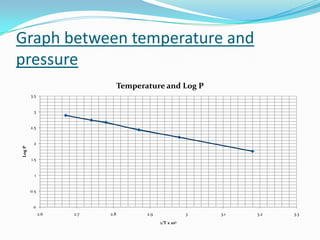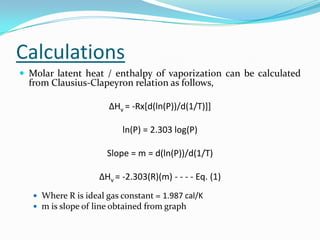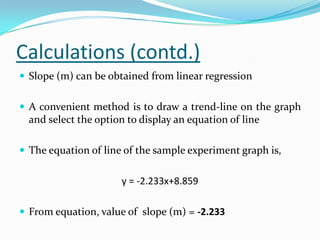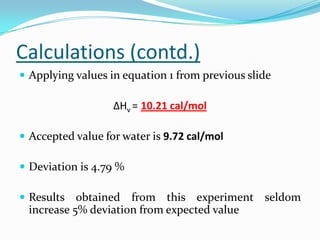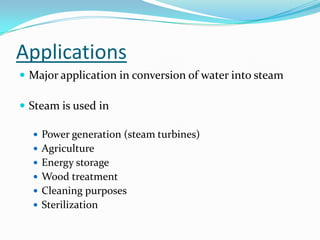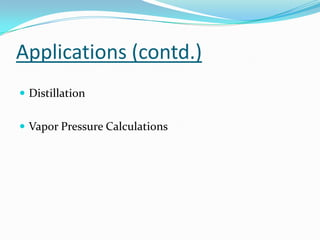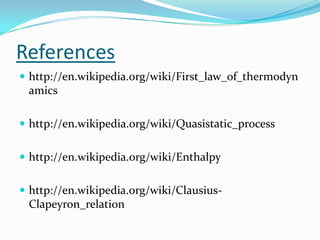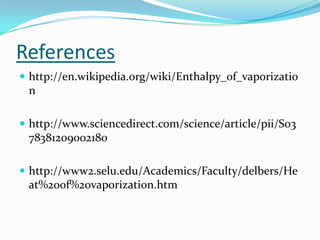1 de 36

### Enthalpy of vaporization of liquid

1. A detailed approach
2. Presented by  Ahmed Asad – CIIT/SP10-BEC-003/LHR  Muhammad Usama – CIIT/SP10-BEC-017/LHR  Mohammad Abubakar – CIIT/SP10-BEC-022/LHR  Noaman Ahmed – CIIT/SP10-BEC-037/LHR  Saad Wazir – CIIT/SP10-BEC-043/LHR  Saim Khan – CIIT/SP10-BEC-044/LHR  Waqar Farooq – CIIT/SP10-BEC-050/LHR
3. Presentation Outline  Restatement of first law of thermodynamics  Definition of enthalpy  Some common enthalpy changes  Enthalpy of vaporization  Characteristics of enthalpy of vaporization  Physical model for vaporization  Experimental determination  Sample readings and calculations  Applications
4. First Law of Thermodynamics  Energy conservation law  Describeschange in internal energy of a thermodynamic system  Clausius’ statement: In a thermodynamic process, the increment in the internal energy of a system is equal to the difference between the increment of heat accumulated by the system and the increment of work done by it.
5. First Law of Thermodynamics (contd.)  In any incremental process, the change in the internal energy is considered due to,  Heat added to the system  Work done by the system dU = dQ - dW
6. First Law of Thermodynamics (contd.)  For a quasistatic process (infinitely slow process), dU = dQ – PdV  NO real process is quasistatic  A quasistatic process ensures that the system will go through a sequence of states that are infinitesimally close to equilibrium (so the system remains in quasistatic equilibrium), in which case the process is typically reversible
7. Quasistatic and Reversibility  Any reversible process is a quasistatic process  Any quasistatic process may not be reversible  Due to heat flow  Due to entropy generation  Example of an irreversible quasistatic process  Compression against a system with a piston subject to friction
8. Enthalpy  Measure of total energy of a thermodynamic system  A state function  Includes  Internal energy (energy required to create a system)  Amount of energy required to establish system’s pressure and volume ΔH = ΔU + Δ(PV)  SI Unit – Joule  Other conventional units – Btu and Calories
9. Why Enthalpy is measured?  Total enthalpy of a system can’t be measured directly  Enthalpy change of a system is measured instead  It is measured to,  Calculate “useful work” obtainable from a closed thermodynamic system under constant pressure  Determine nature of reaction e.g., exothermic or endothermic
10. Enthalpy is not necessarily heat !!  Enthalpy is sometimes described as heat content of a system  Heat is defined as thermal energy in transit  For the description that enthalpy is in-fact heat to be valid, no energy exchange must occur with environment other than heat or expansion work
11. Common Enthalpy Changes  Enthalpy of reaction  Enthalpy of formation  Enthalpy of combustion  Enthalpy of neutralization  Enthalpy of solution  Enthalpy of vaporization  Enthalpy of sublimation
12. Vaporization  Phase transition from liquid phase to gas phase  Two types  Evaporation  Occurs at temperatures below boiling temperature  Usually occurs on surface  Boiling  Occurs at or above boiling temperature  Occurs below the surface
13. Enthalpy of Vaporization (EOV)  Enthalpy change required to completely change the state of one mole of substance between liquid and gaseous states  Energy required to transform a given quantity of a substance from a liquid into a gas at a given pressure  Usually measured at boiling point of a substance
14. Characteristics of Enthalpy of Vaporization  It is temperature dependent  EOV decreases with increase in temperature  EOV diminishes completely at critical temperature beyond which liquid and vapor phase no longer co- exist  Units – J/mol or kJ/mol, kJ/kg, Btu/lb, kcal/mol
15. Characteristics of Enthalpy of Vaporization (contd.)  Enthalpy of condensation is same as enthalpy of vaporization but with opposite sign  Enthalpy change of vaporization is always positive  Enthalpy change of condensation is always negative
16. Temperature dependence of EOV
17. Physical model for vaporization  Proposed by professor Jozsef Garai, Florida International University, USA  Energy required to free an atom from liquid is equivalent to energy required to overcome surface resistance of liquid  This model states, Latent heat = (Max. surface area) x (Surface tension) x (No. of atoms in liquid)
18. Physical model for vaporization (Diagrammatic representation)
19. Experimental determination
20. Apparatus  Round bottom boiling flask  Distillation condenser or multiple condensers  Heat source (a burner or a heating mantle)  A vacuum gauge (Bourdon type gauge)  Aspirator or trapped vacuum pump  Pressure-regulating device (a needle valve that is part of a Bunsen burner base)  Thermometer
21. Basic Goal  To determine boiling point of the liquid (water) under study at different pressure values  To determine enthalpy of vaporization using the Clausius-Clapeyron relation
22. Clausius-Clapeyron relation  A relation used to characterize a discontinuous phase transition between two phases of a single constituent  On a P-T diagram, line separating two phases is known as coexistence curve  This relation gives the slope of the tangents to this curve
23. Clausius-Clapeyron relation (contd.)  General form dP/dT = L/TΔV  Where  dP/dT is slope of tangent to coexistence curve at any point  L is latent specific heat  T is temperature  ΔV is specific volume change of phase transition  For transitions between a gas and condensed phase, the expression may be rewritten as, ln(P) = (-L/R) x (1/T) + C
24. Procedure  Maintain lowest possible pressure by closing bleed valve  Set water flow to the aspirator at maximum level to provide highest vacuum  Place few boiling stones in round bottom flask to minimize bumping
25. Procedure (contd.)  Temperature increases until boiling starts  When boiling occurs, allow the thermometer reading to stabilize for 1 to 2 minutes and note the temperature  Note the pressure reading on the manometer at this temperature
26. Procedure (contd.)  Increase the pressure of the vessel by slightly opening the bleed valve  Repeat the same procedure as described previously and then increase pressure again  Take at least five readings and plot a graph between reciprocal of temperature and log of pressure difference
27. Sample Readings Temp (°C) h (mm Hg) Temp (K) (1/T) x 103 P (mm Hg) Log P (1/K) (P = 768 –h) 41.5 710 314.5 3.18 58.0 1.77 62.5 610 335.2 2.98 158 2.20 75.0 500 348.0 2.87 268 2.43 86.5 300 359.5 2.78 468 2.67 92.2 220 365.2 2.74 548 2.74 101.0 0 374.0 2.67 768 2.89
28. Graph between temperature and pressure Temperature and Log P 3.5 3 2.5 2 Log P 1.5 1 0.5 0 2.6 2.7 2.8 2.9 3 3.1 3.2 3.3 1/T x 103
29. Calculations  Molar latent heat / enthalpy of vaporization can be calculated from Clausius-Clapeyron relation as follows, ΔHv = -Rx[d(ln(P))/d(1/T)]] ln(P) = 2.303 log(P) Slope = m = d(ln(P))/d(1/T) ΔHv = -2.303(R)(m) - - - - Eq. (1)  Where R is ideal gas constant = 1.987 cal/K  m is slope of line obtained from graph
30. Calculations (contd.)  Slope (m) can be obtained from linear regression  A convenient method is to draw a trend-line on the graph and select the option to display an equation of line  The equation of line of the sample experiment graph is, y = -2.233x+8.859  From equation, value of slope (m) = -2.233
31. Calculations (contd.)  Applying values in equation 1 from previous slide ΔHv = 10.21 cal/mol  Accepted value for water is 9.72 cal/mol  Deviation is 4.79 %  Results obtained from this experiment seldom increase 5% deviation from expected value
32. Applications  Major application in conversion of water into steam  Steam is used in  Power generation (steam turbines)  Agriculture  Energy storage  Wood treatment  Cleaning purposes  Sterilization
33. Applications (contd.)  Distillation  Vapor Pressure Calculations
34. References  http://en.wikipedia.org/wiki/First_law_of_thermodyn amics  http://en.wikipedia.org/wiki/Quasistatic_process  http://en.wikipedia.org/wiki/Enthalpy  http://en.wikipedia.org/wiki/Clausius- Clapeyron_relation
35. References  http://en.wikipedia.org/wiki/Enthalpy_of_vaporizatio n  http://www.sciencedirect.com/science/article/pii/S03 78381209002180  http://www2.selu.edu/Academics/Faculty/delbers/He at%20of%20vaporization.htm
36. QUESTIONS ARE WELCOMED !!

### Notas del editor

1. Noaman StartThe first law of thermodynamics is a specialized version of law of conservation of energy for thermodynamicsThe first explicit statement of this law was given by Rudolf Clausius in 1850, and in terms of internal energy the statement was (SLIDE)
2. where dQand dW are infinitesimal amounts of heat supplied to the system and work done by the system, respectively. Note that the minus sign in front of indicates that a positive amount of work done by the system leads to energy being lost from the system. This is the sign convention used in many textbooks on physics.(An alternate sign convention is to consider the work performed on the system by its surroundings. This leads to a change in sign of the work, so that . This is the convention adopted by many modern textbooks of physical chemistry, such as those by Peter Atkins and Ira Levine.)
3. In practice, such processes can only be approximated by performing them infinitesimally slowly.A quasistatic process ensures that the system will go through a sequence of states that are infinitesimally close to equilibrium (so the system remains in quasistatic equilibrium), in which case the process is typically reversible.
4. Any reversible process is necessarily a quasistatic one. However, some quasistatic processes are irreversible, if there is heat flowing (in or out of the system) or if entropy is being created in some other way. An example of a quasistatic process that is not reversible is a compression against a system with a piston subject to friction — Although the system is always in thermal equilibrium, the friction ensures the generation of dissipative entropy, which directly goes against the definition of reversible.
6. The total enthalpy of a system cannot be measured directly; the enthalpy change of a system is measured instead.
7. Adding or removing energy through heat is one of only two ways to change the enthalpyEnthalpy is nothing more than heat &quot;stored&quot; by the system, provided the given restrictions are adhered toEnthalpy also changes when the pressure of the environment is altered, even if no energy is exchanged as heatEnthalpy changes when energy is transferred into or out of the system through a means other than heat or expansion work, such as through external fields or stirring
8. Enthalpy of reaction, defined as the enthalpy change observed in a constituent of a thermodynamic system when one mole of substance reacts completely. Enthalpy of formation, defined as the enthalpy change observed in a constituent of a thermodynamic system when, one mole of a compound is formed from its elementary antecedents. Enthalpy of combustion, defined as the enthalpy change observed in a constituent of a thermodynamic system, when one mole of a substance combusts completely with oxygen.Enthalpy of neutralization, defined as the enthalpy change observed in a constituent of a thermodynamic system, when one mole of water is produced when an acid and a base react.Enthalpy of solution, defined as the enthalpy change observed in a constituent of a thermodynamic system, when one mole of an solute is dissolved completely in an excess of solvent.Enthalpy of vaporization, defined as the enthalpy change required to completely change the state of one mole of substance between liquid and gaseous states. Enthalpy of sublimation, defined as the enthalpy change required to completely change the state of one mole of substance between solid and gaseous states.
9. Vaporization is a phase transition from a liquid phase to a gas phase